# High School Physics : Understanding Types of Waves

## Example Questions

### Example Question #1 : Waves

Order the following electromagnetic waves from the longest wavelength to the shortest: Gamma Rays, Infrared, Microwaves, Radio Waves, Ultraviolet, Visible Light, X-Rays

Gamma Rays, X-Rays, Ultraviolet, Visible Light, Infrared, Microwaves, Radio Waves

Radio Waves, Gamma Rays, Microwaves, Infrared, Visible Light, X-Rays, Ultraviolet

Gamma Rays, Infrared, Microwaves, Radio Waves, Ultraviolet, Visible Light, X-Rays

Visible Light, Infrared, Microwaves, Gamma Rays, X-Rays, Ultraviolet, Radio Waves

Radio Waves, Microwaves, Infrared, Visible Light, Ultraviolet, X-Rays, Gamma Rays

Radio Waves, Microwaves, Infrared, Visible Light, Ultraviolet, X-Rays, Gamma Rays

Explanation:

Radio waves have the smallest frequency and longest wavelength. This is why they are not dangerous. Microwaves have the next longest wavelength and are what are used to warm up cold foods. Infrared waves are the next longest wavelength and border the red light on the visible spectrum. Infrared waves are used in remote controls and night vision. Visible light is next and is what we can see with our eyes. Next is ultraviolet, which borders the violet light on the visible spectrum. These are the damaging rays by the sun and are essentially “super-violet” rays. Next is X-rays. These have very high frequencies and are dangerous in high quantities. Gamma rays are the shortest wavelength and the highest frequency and the most dangerous. These cosmic rays often come from stars and other celestial objects.

### Example Question #1 : Waves

Two waves are traveling toward each other along a rope. When they meet, the waves

pass through each other

disappear

bounce off each other

pass through each other

Explanation:

When waves interfere with one another, they pass through each, and others can undergo either constructive or destructive interference.  In constructive interference, waves add together to produce a briefly more massive wave.  In destructive interference, waves subtract from each other to create a smaller wave.  However, once the waves move past one another, they will return to their original shape and size.

### Example Question #6 : Waves

A wave transports

matter but not energy

energy but not matter

both energy and matter

energy but not matter

Explanation:

Waves carry energy along the path displacing the matter for a brief period. However, the matter does not travel along the wave and instead will return to its natural rest position once the wave moves past it. For example, an ocean wave can travel many miles without displacing the entire ocean.

### Example Question #7 : Waves

In seismology, the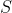wave is a transverse wave. As anwave travels through the Earth, the relative motion between thewave and the particles is

Perpendicular

Parallel

First parallel, then perpendicular

First perpendicular, then parallel

Perpendicular

Explanation:

Transverse waves are waves whose particles travel perpendicular to the direction that the wave itself is traveling. Electromagnetic waves are another example of transverse waves.

### Example Question #8 : Waves

A radio station broadcasts at a frequency of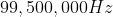. If the broadcast is an electromagnetic wave, then what is its wavelength?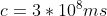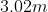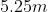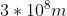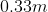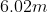Explanation:

The relationship between wavelength and frequency is given by the equation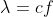where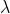is the wavelength,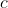is the speed of light, and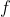is frequency.

We are given the values for frequency and the speed of light, allowing us to solve for the wavelength.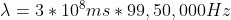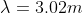### Example Question #1 : Waves

In a vacuum, the velocity of all electromagnetic waves

Is zero

is nearly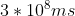depends on the amplitude

depends on the frequency

is nearlyExplanation:

Electromagnetic waves all travel at the same speed which is the speed of light.  The speed of light in a vacuum is nearly### Example Question #10 : Waves

Which of the following waves require a medium to travel?

Light Waves

Sound Waves

Microwaves

Sound Waves

Explanation:

Electromagnetic waves are the only type of wave that does not require a medium to travel. Light, radio, and microwaves are examples of electromagnetic waves. Sound does require a medium to travel. In a vacuum, soundwaves cannot travel as there is no air to compress.

### Example Question #11 : Waves

In seismology, the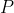wave is a longitudinal wave. As anwave travels through the Earth, the relative motion between thewave and the particles is

First parallel, then perpendicular

Perpendicular

First perpendicular, then parallel

Parallel

Parallel

Explanation:

Longitudinal waves are waves whose particles travel parallel to the direction that the wave itself is traveling. Sound waves are another example of longitudinal waves.

### Example Question #12 : Waves

A student attaches one end of a Slinky to the top of a table. She holds the other end in her hand, stretches it to a length, and then moves it back and forth to send a wave down the Slinky. If she next moves her hand faster while keeping the length of the Slinky the same, how does the wavelength down the slinky change?

It increases

It stays the same

It decreases

It decreases

Explanation:

The speed of the wave along the Slinky depends on the mass of the Slinky itself and the tension caused by stretching it. Since both of these things have not changed, the wave speed remains constant.

The wave speed is equal to the wavelength multiplied by the frequency.Since she is moving her hand faster, the frequency has increased. Since the velocity has not changed, an increase in the frequency would decrease the wavelength.

### Example Question #12 : Waves

Sound waves are

Longitudinal waves characterized by both the displacement of air molecules and pressure differences

Transverse waves characterized by the displacement of air molecules

Longitudinal waves characterized by the displacement of air molecules

Longitudinal waves characterized by pressure differences